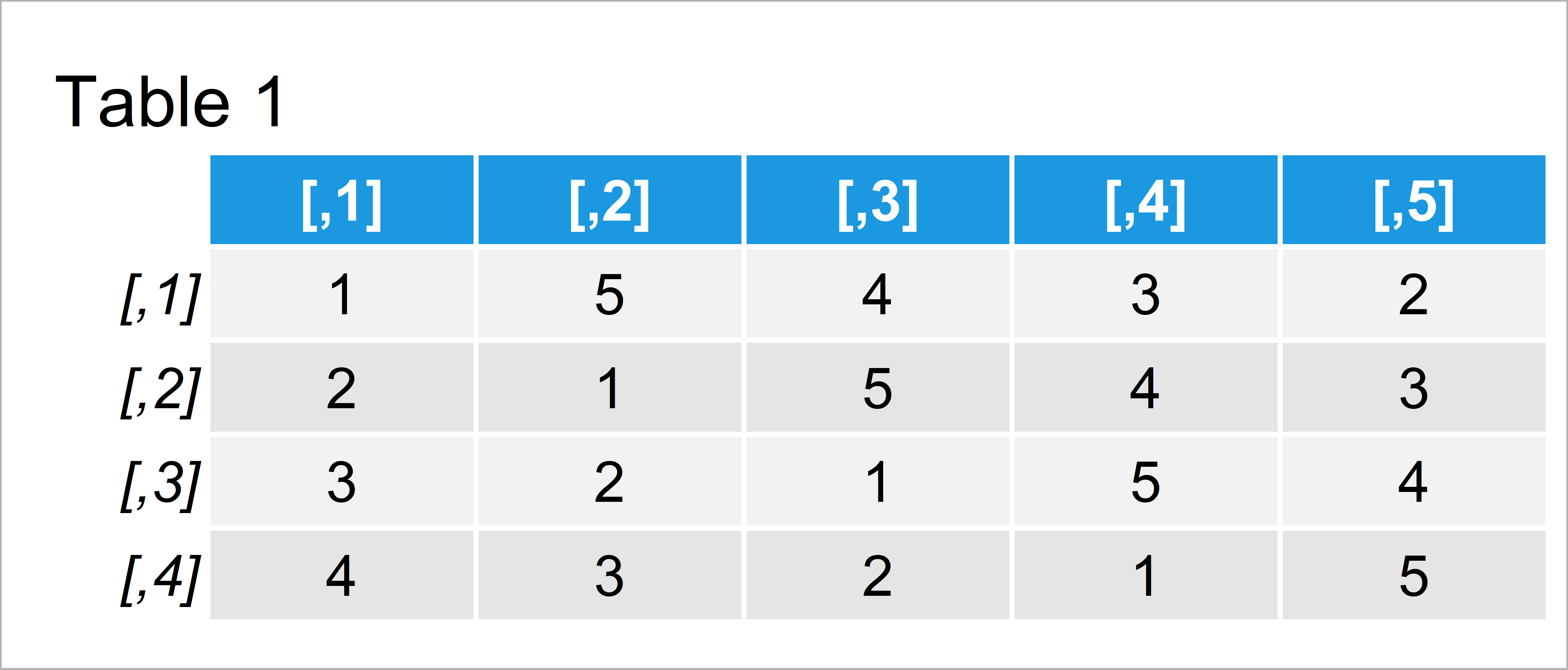# Return Index Position of Element in Matrix Using which() Function in R (Example)

In this tutorial, I’ll illustrate how to find the index positions of certain elements in a matrix using the which() function in R.

So let’s dig in.

## Introducing Example Data

Let’s first construct some example data:

```mat <- matrix(1:5,                # Create example matrix
ncol = 5,
nrow = 4)
mat                               # Print example matrix```Have a look at the table that got returned after running the previously shown R programming code. It shows that our exemplifying matrix contains four observations and five columns.

## Example 1: Return Vector of Positions Using which() Function with Default Specifications

In this example, I’ll show how to find the positions of a specific value after converting our matrix to a vector object.

Have a look at the following R syntax:

```which(mat == 4)                   # Apply which function with default specifications
#   4  9 14 19```

The RStudio console has returned a vector of indices for the value 4. It indicates that the value four appears at the 4th, 9th, 14th, and 19th position of our matrix.

However, the previous result is difficult to interpret, since it doesn’t show in which rows or columns our value appears.

For that reason, I’ll show you a little trick in the next example that returns the row and column indices of a particular value. Keep on reading!

## Example 2: Return Matrix of Row & Column Positions Using arr.ind Argument of which() Function

In this example, I’ll show how to identify the row and column indices of a certain element in a matrix.

For this, we use the which function again, but this time we specify the arr.ind to be equal to TRUE:

```mat_pos <- which(mat == 4,        # Set arr.ind = TRUE
arr.ind = TRUE)
mat_pos                           # Print position matrix of value 4```The output of the previous R syntax is shown in Table 2: We have created a matrix showing the row and column indices of the value 4 in our example matrix.

## Video & Further Resources

In case you need more information on the R syntax of this article, I recommend having a look at the following video of my YouTube channel. In the video, I’m explaining the R programming codes of this post in a live session:

Please accept YouTube cookies to play this video. By accepting you will be accessing content from YouTube, a service provided by an external third party.If you accept this notice, your choice will be saved and the page will refresh.

Besides that, you might want to read the related articles on my homepage. You can find a selection of tutorials about matrices here.

At this point you should know how to apply the which function to matrices in R. Please tell me about it in the comments section, in case you have additional comments and/or questions.

Subscribe to the Statistics Globe Newsletter